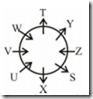Tuesday, 1 November 2016

Reasoning Ability Quiz for IBPS Clerk quiz – 2

Directions (Q. 1-5): In each question below are given  two  statements  followed  by  two  conclusions numbered I and II. You have to take the given statements to  be  true  even  if  they  seem  to  be  at  variance  with commonly known facts. Read all the conclusions and then decide which of the given conclusions logically follows from  the  given  statements,  disregarding  commonly known facts. Give answer

1) if only conclusion I follows.
2) if only conclusion II follows.
3) if either conclusion I or II follows.
4) if neither conclusion I nor II follows.
5) if both conclusions I and II follow

1. Statements:
Some buses are trucks.
No truck is a jeep.

Conclusions:
I. Some jeeps are trucks.
II. Some buses are not jeeps.

2. Statements:
All sofas are beds.
Some beds are tables.

Conclusions:
I. At least some  sofas being tables is a possibility.
II. Some tables are beds.

3. Statements:
Some papers are books.
All books are pages.

Conclusions:
I. Some pages are not papers.
II. No book is paper.

4. Statements:
No desk is a shelf.
Some shelves are stools.

Conclusions:
I. No stool is a desk.
II. Some stools are not desks.

5. Statements:
No clock is a laptop.

Conclusions:
I. Some radios are not laptops.

Directions  (Q.  6-10):  In  these  questions,  a relationship between different elements is shown in the statement(s).  The  statements  are  followed  by  two conclusions. Give answer .

1) if only conclusion I is true.
2) if only conclusion II is true.
3) if either conclusion I or II is true.
4) if neither conclusion I nor II is true.
5) if both conclusions I and II are true

6. Statements: T ?S = R > Q > P
Conclusions:
I.P ?T
II.T > Q

7. Statement: P ?O < N > M ?L
Conclusions:
I.O > M
II.N  ?L

8. Statement: A ?B = C > D  ?E
Conclusions:
I.E > C
II.D < A

9. Statements: H > J = K, K > L > T, T ?V
Conclusions:
I.H > L
II.J > V

10. Statements: U ?V < W, W = N < L
Conclusions:
I.L > V
II.U ?N

Directions (Q. 11-15): Study the following information carefully to answer the given questions:

Eight members of a club are sitting around a circular table, viz Z, Y, X, W, V, U, T and S, but not necessarily in the same order. Four of them are not facing the centre. W is facing the centre and is third to the right of Z, who is third to the right of U, who is facing the centre. X is fourth to the left of T. S is third to the right of V, who is not a neighbour of T. V sits second to the right of X. Y is on the immediate right of T.

11. What is S’s position with respect to Y?
1) Third to the left
2) Second to the right
3) Second to the left
4) Either 2) or 3)
5) None of these

12. Who is second to the left of V?
1) W
2) X
3) Y
4) T
5) None of these

13. Which of the following pairs are neighbours of T?
1) W, Y
2) U, S
3) Z, W
4) Can’t be determined
5) None of these

14. Four of the following five are alike in a certain way and so  form  a  group.  Which  is  the  one  that  does  not belong to that group?
1) W
2) Y
3) V
4) Z
5) U

15. Which of the following statements is/are true?
1) Z is not facing the centre.
2) Y is second to the right of W.
3) T is facing the centre
4) Both 1) and 2) are true
5) None of these

1.2; No truck is a jeep  ? conversion  ? No jeep  is  a  truck.  Hence,  conclusion  I  does  not follow. Again, Some buses  are trucks (I) + No truck is a jeep (E) = I + E = O = Some buses are not jeeps.  Hence,  conclusion  II  follows.

2.5; All sofas are beds (A) + Some beds are tables  (I) = A  +  I  =  No  conclusion.  But  the possibility exists. Hence, conclusion I  follows. Again,  Some  beds  are  tables  ?  conversion ?  Some  tables  are  beds.  Hence, conclusion II also follows.

3.4; Some  papers are books  (I) + All books are pages (A) = I + A  = I  = Some papers  are pages.  Hence,  conclusion  I does  not  follow. Again, Some papers  are books  ? conversion ? Some books  are  papers. Hence, conclusion II  does  not  follow.

4.2; No desk is a shelf (E) + Some shelves are stools (I) = E + I  = O* = Some stools are not  desks. Hence,  conclusion II  follows  but  I does  not  follow.

5.5; Some radios are clocks  (I) +  No  clock is a laptop (E) = I + E = O = Some radios are not  laptops.  Hence,  conclusion  I  follows. Again, Some radios are clocks  ? conversion ? Some clocks are radios. Hence, conclusion II  follows.

6.2; T  ?S = R > Q > P Thus, T >  P is true. Hence, conclusion I  does not  hold  true. But, T > Q is true. Hence, conclusion II holds true

7. 4

8. 2

9.1

10. 1

(11-15)11.2

12.4

13.1

14.2

15. 5